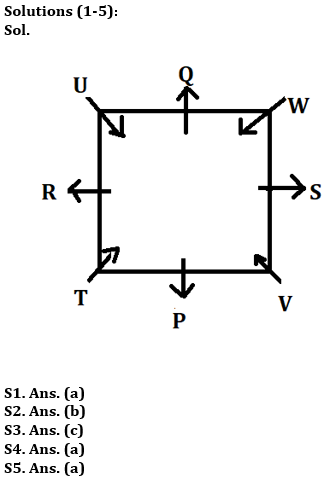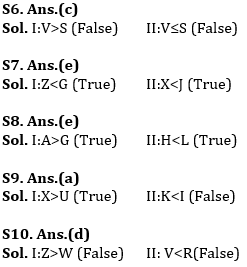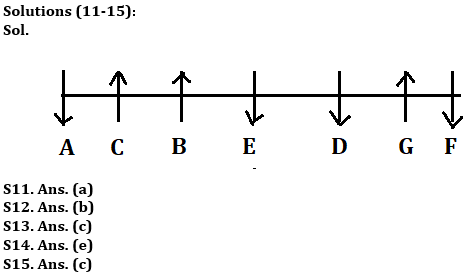Latest Banking jobs   »   Reasoning Ability Quiz For ESIC- UDC,...

# Reasoning Ability Quiz For ESIC- UDC, Steno, MTS Prelims 2022- 11th January

Directions (1-5): Study the following information carefully and answer the questions given below:
Eight persons P, Q, R, S, T, U, V and W are sitting on a square table such that some persons are sitting on the corners and some persons are sitting in middle of the table. The one who sits at the corner are facing towards the centre of the table and the one who sits at the middle are facing outside the centre of table but not necessarily in the same order. Q and V are not immediate neighbours of each other . T sits immediate right of P. P is facing outside the centre table and one person sits between P and R . Two persons sit between R and V . S sits immediate right of V. One person sits between V and W .W and P are not immediate neighbours of each other. U and Q are immediate neighbours of each other. Q is not facing the centre of the table.

Q1. Who among the following person sits immediate left of T?
(a) R
(b) U
(c) Q
(d) W
(e) None of these

Q2.Four of the following five are alike in a certain way and hence form a group find the one which does not belong to that group?
(a) T
(b) P
(c) V
(d) W
(e) U

Q3. If P and Q interchange their position then who sits immediate right of Q ?
(a) W
(b) S
(c) T
(d) R
(e) None of these

Q4. Who among the following person sits immediate left of W?
(a) S
(b) V
(c) U
(d) P
(e) None of these

Q5. Who among the following person is facing U?
(a) V
(b) S
(c) W
(d) T
(e) None of these

Directions (6-10): In each of the question, relationships between some elements are shown in the statements. These statements are followed by conclusions numbered I and II. Read the statements and give the answer.
(a) If only conclusion I follows.
(b) If only conclusion II follows.
(c) If either conclusion I or II follows.
(d) If neither conclusion I nor II follows.
(e) If both conclusions I and II follow.

Q6. Statements: C>V≥B<N<M>A>S>D>F>G
Conclusion:
I: V>S
II: V≤S

Q7. Statements: Z<X<C≤V;B>C<G<H≤J
Conclusion:
I: Z<G
II: X<J

Q8. Statements: A>S>D>F>G<H<J<K<L
Conclusion:
I: A>G
II: H<L

Q9. Statements: X>C>H≥J≥K;H>Y≥U<I<O
Conclusion:
I:X>U
II: K<I

Q10. Statements: Z>X>C<V; X>A>S<D<F; D>Q<W<R
Conclusion:
I:Z>W
II: V<R

Directions (11-15): Study the following information carefully and answer the questions given below:
Seven persons A, B, C, D, E, F, and G are sitting in a row some are facing north and some are facing south direction but not necessarily in the same order. The number of persons facing south is more than the number of person facing north direction. A sits immediate left of C and A sits at one of the extreme end of the row. Number of persons sitting to the left of E is same as the number of persons sitting to the right of E. A sits to the right of E. One person sits between D and F and F is not immediate neighbour of E. G sits to the right of C but not immediate right. D is facing the same direction as E. A and C are facing opposite direction to each other. The persons sitting at the end are facing the same direction . The person who sits at the immediate right of E is facing north direction. B and G is facing the same direction.

Q11.Who among the following person sits exactly between C and G ?
(a) E
(b) A
(c) D
(d) F
(e) None of these

Q12.What is the position of D with respect to B ?
(a) Immediate left
(b) Second to the right
(c) Second to the left
(d) Immediate right
(e) None of these

Q13. How many persons sit between G and F ?
(a) One
(b) Two
(c) None
(d) Three
(e) None of these

Q14.Four of the following five are alike in a certain way and hence form a group find the one which does not belong to that group ?
(a) A
(b) E
(c) D
(d) F
(e) G

Q15. If B and F interchange their position then who sits immediate right of B ?
(a) C
(b) D
(c) G
(d) A
(e) None of these

Solutions#### Congratulations!Union Budget 2023-24: Free PDF